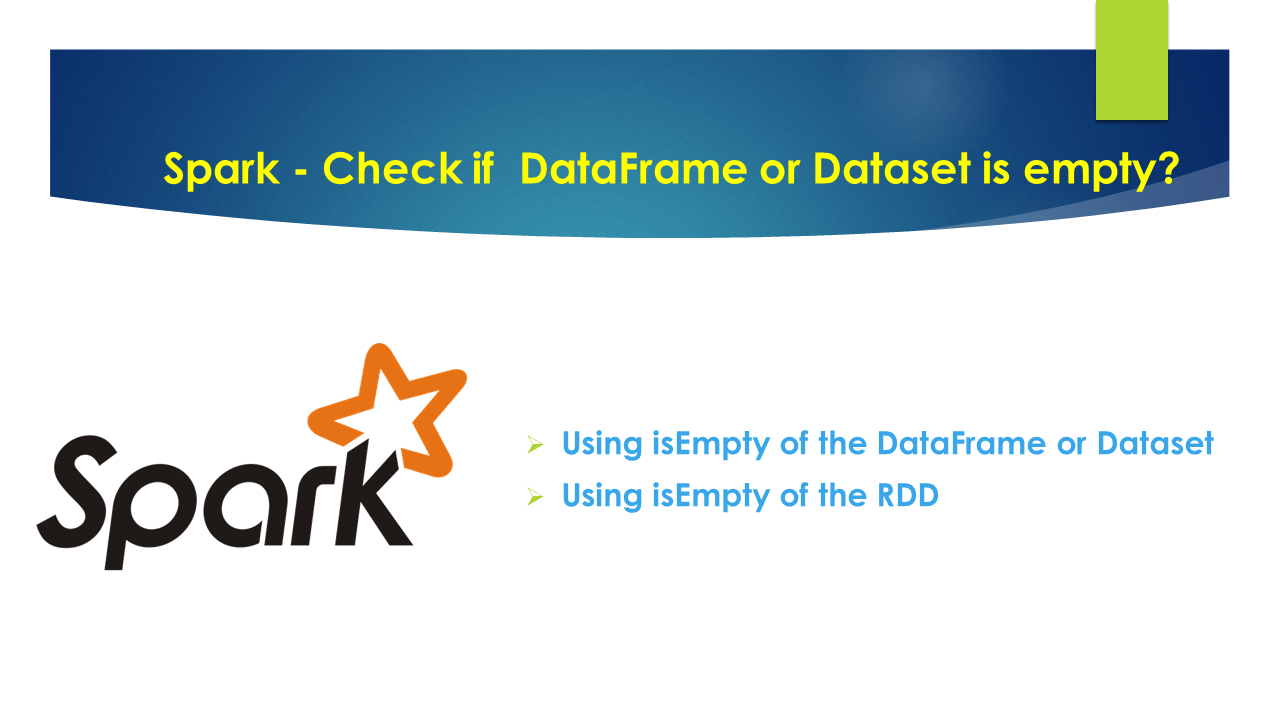# Spark – Check if DataFrame or Dataset is empty?

In Spark, `isEmpty` of the DataFrame class is used to check if the DataFrame or Dataset is empty, this returns `true `when empty otherwise return `false`. Besides this, Spark also has multiple ways to check if DataFrame is empty. In this article, I will explain all different ways and compare these with the performance see which one is best to use.

First, let’s create an empty DataFrame

``````
val df = spark.emptyDataFrame
``````

## Using isEmpty of the DataFrame or Dataset

`isEmpty` function of the DataFrame or Dataset returns true when the dataset empty and false when it’s not empty.

``````
df.isEmpty
``````

Alternatively, you can also check for DataFrame empty.

``````
``````

Note that calling `df.head()` and `df.first()` on empty DataFrame returns `java.util.NoSuchElementException: next on empty iterator` exception.

You can also use the below but this is not efficient as above hence use it wisely when you have a small dataset. df.count calculates the count from all partitions from all nodes hence do not use it when you have millions of records.

``````
print(df.count > 0)
``````

## 1. Using isEmpty of the RDD

This is most performed way of check if DataFrame or Dataset is empty.

``````
// Using isEmpty of the RDD
df.rdd.isEmpty()
``````

## Conclusion

In Summary, we can check the Spark DataFrame empty or not by using isEmpty function of the DataFrame, Dataset and RDD. if you have performance issues calling it on DataFrame, you can try using `df.rdd.isempty`

Happy Learning !!

### Naveen (NNK)

Naveen (NNK) is a Data Engineer with 20+ years of experience in transforming data into actionable insights. Over the years, He has honed his expertise in designing, implementing, and maintaining data pipelines with frameworks like Apache Spark, PySpark, Pandas, R, Hive and Machine Learning. Naveen journey in the field of data engineering has been a continuous learning, innovation, and a strong commitment to data integrity. In this blog, he shares his experiences with the data as he come across. Follow Naveen @ @ LinkedIn